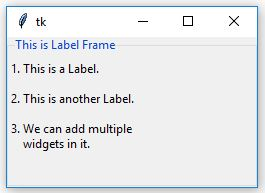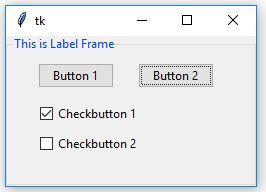# Python Tkinter | Create LabelFrame and add widgets to it

Tkinter is a Python module which is used to create GUI (Graphical User Interface) applications. It is a widely used module which comes along with the Python. It consists of various types of widgets which can be used to make GUI more user-friendly and attractive as well as functionality can be increased.

LabelFrame can be created as follows:

```-> import tkinter
-> create root
-> create LabelFrame as child of root
```
`label_frame = ttk.LabelFrame(parent, value = options, ...)`

Code #1: Creating LabelFrame and adding a message to it.

 `# Import only those methods ` `# which are mentioned below, this way of ` `# importing methods is efficient ` `from` `tkinter ``import` `Tk, mainloop, LEFT, TOP ` `from` `tkinter.ttk ``import` `*` ` `  `# Creating tkinter window with fixed geometry ` `root ``=` `Tk() ` `root.geometry(``'250x150'``) ` ` `  `# This will create a LabelFrame ` `label_frame ``=` `LabelFrame(root, text ``=` `'This is Label Frame'``) ` `label_frame.pack(expand ``=` `'yes'``, fill ``=` `'both'``) ` ` `  `label1 ``=` `Label(label_frame, text ``=` `'1. This is a Label.'``) ` `label1.place(x ``=` `0``, y ``=` `5``) ` ` `  `label2 ``=` `Label(label_frame, text ``=` `'2. This is another Label.'``) ` `label2.place(x ``=` `0``, y ``=` `35``) ` ` `  `label3 ``=` `Label(label_frame, ` `           ``text ``=` `'3. We can add multiple\n    widgets in it.'``) ` ` `  `label3.place(x ``=` `0``, y ``=` `65``) ` ` `  `# This creates an infinite loop which generally ` `# waits for any interrupt (like keyboard or ` `# mouse) to terminate ` `mainloop() `

Output:Code #2: Adding Button and CheckButton widgets inside LabelFrame.

 `# Import only those methods ` `# which are mentioned below, this way of ` `# importing methods is efficient ` `from` `tkinter ``import` `Tk, mainloop, LEFT, TOP ` `from` `tkinter.ttk ``import` `*` ` `  `# Creating tkinter window with fixed geometry ` `root ``=` `Tk() ` `root.geometry(``'250x150'``) ` ` `  `# This will create a LabelFrame ` `label_frame ``=` `LabelFrame(root, text ``=` `'This is Label Frame'``) ` `label_frame.pack(expand ``=` `'yes'``, fill ``=` `'both'``) ` ` `  `# Buttons ` `btn1 ``=` `Button(label_frame, text ``=` `'Button 1'``) ` `btn1.place(x ``=` `30``, y ``=` `10``) ` `btn2 ``=` `Button(label_frame, text ``=` `'Button 2'``) ` `btn2.place(x ``=` `130``, y ``=` `10``) ` ` `  `# Checkbuttons ` `chkbtn1 ``=` `Checkbutton(label_frame, text ``=` `'Checkbutton 1'``) ` `chkbtn1.place(x ``=` `30``, y ``=` `50``) ` `chkbtn2 ``=` `Checkbutton(label_frame, text ``=` `'Checkbutton 2'``) ` `chkbtn2.place(x ``=` `30``, y ``=` `80``) ` ` `  `# This creates infinite loop which generally ` `# waits for any interrupt (like keyboard or ` `# mouse) to terminate ` `mainloop() `

Output:Note: One can also add another LabelFrame inside another LabelFrame, as well as one can do styling of any LabelFrame like we do the styling of other widgets.

My Personal Notes arrow_drop_upCheck out this Author's contributed articles.

If you like GeeksforGeeks and would like to contribute, you can also write an article using contribute.geeksforgeeks.org or mail your article to contribute@geeksforgeeks.org. See your article appearing on the GeeksforGeeks main page and help other Geeks.

Please Improve this article if you find anything incorrect by clicking on the "Improve Article" button below.

Article Tags :

Be the First to upvote.

Please write to us at contribute@geeksforgeeks.org to report any issue with the above content.